# DAV Class 5 Maths Chapter 16 Brain Teasers Solutions

The DAV Class 5 Maths Solutions and DAV Class 5 Maths Chapter 16 Brain Teasers Solutions of Triangles offer comprehensive answers to textbook questions.

## DAV Class 5 Maths Ch 16 Brain Teasers Solutions

Question 1.
(a) Which of the following cannot be the angles of a triangle?
(i) 70°, 50°, 60°
(ii) 90°, 45°, 45°
(iii) 100°, 40°, 30°
(iv) 100°, 50°, 30°
Solution:
(iii) 100°, 40°, 30°
∵ 100° + 40° + 30° = 170°

(b) The third angle of a right-angled triangle whose one angle is 50° is-
(i) 40°
(ii) 130°
(iii) 55°
(iv) 45°
Solution:
(i) 40°
∴ 90° + 50° + x° = 180°
⇒ x° = 180 – 140°
⇒ x° = 40°

(c) There are ________ triangles in the given figure.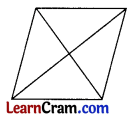(i) 4
(ii) 6
(iii) 8
(iv) 5
Solution:
(iii) 8(d) Which of the following can be the third side of a triangle whose two sides are 7 cm and 9 cm respectively?
(i) 16 cm
(ii) 18 cm
(iii) 14 cm
(iv) 17 cm
Solution:
(iii) 14 cm
As sum of the two sides must be greater than the third side.

(e) In the given figure ∆ABC is an equilateral triangle. What type of triangle is ∆ACD?(i) Scalene triangle
(ii) Right-angled triangle
(iii) Equilateral triangle
(iv) Isosceles triangle
Solution:
(iii) Equilateral triangle

Question 2.
A closed figure is made of three line segments what is the figure called?
Solution:
Triangle

Question 3.
Name the vertices, sides, and angles of a triangle.Solution:
Vertices = X, Y, Z
Sides = XY, YZ, XZ
Angles = ∠X or ∠YXZ, ∠Y or ∠XYZ, ∠Z or ∠XZY

Question 4.
How many triangles are there in each?
(a)Solution:
5 triangles

(b)Solution:
6 trianglesQuestion 5.
Find the missing angle. State the kind of triangle.
(a)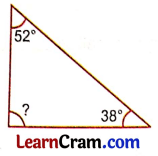Solution:
Let the missing angle be x°
52° + 38° + x° = 180° (sum of angles of Δ is 180°)
⇒ 90° + x° = 180°
⇒ x = 90°

(b)Solution:
Let the missing angle be x°
75° + 65° + x° = 180° (Sum of angles of Δ is 180°)
⇒ 140° + x° = 180°
⇒ x° = 180° – 140°
⇒ x° = 40°

Question 6.
State the kind of triangle.
(a)Solution:
Isosceles triangle

(b)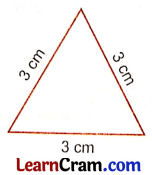Solution:
Equilateral triangle

Question 7.
Can you construct a triangle with angles 70°, 35°, 85°? Why.
Solution:
To construct a triangle we must have the sum of three angles 180°.
70° + 35° + 85° = 190°
But in this case, the sum is greater than 180° so is not possible to construct a triangle.

Question 8.
Can you construct a triangle with line segments of lengths 11 cm, 7 cm, and 8 cm? Give reasons.
Solution:
To construct a triangle, the sum of two sides of a triangle must be greater than the third side.
So 11 cm + 7 cm = 18 cm (sum of two sides)
One of the sides is 8 cm
18 cm > 8 cm possible.
Sum of two sides = 7 cm + 8 cm = 15 cm
Third side = 11 cm
15 cm > 11 cm possible.
Sum of other two sides = 11 cm + 8 cm = 19 cm
Third side = 7 cm
19 cm > 7 cm possible
So we can construct a triangle.Question 9.
State True or False.
(a) A triangle can have two right angles. ________
Solution:
False

(b) An obtuse-angled triangle has one obtuse angle and two acute angles. ________
Solution:
True

(c) The sum of the lengths of any two sides of a triangle is equal to the third side. ________
Solution:
False

(d) The sides of an equilateral triangle are of equal length. ________
Solution:
True

Question 1.
Name the closed figure made of three line segments. How many vertices, sides, and angles it has?
Solution:
Triangle: A triangle has three vertices, three sides, and three angles.

Question 2.
Write two properties of a triangle.
Solution:
Two properties are given below:

• The sum of measures of three angles of a triangle is always 180°.
• The sum of the two sides of a triangle is always greater than the third side.

Question 3.
How many ways a triangle is classified?
Solution:
Triangles are classified in two ways.

• According to sides
• According to angles

Question 4.
Classify the following ∆ as acute, obtuse, and right triangle.
(a)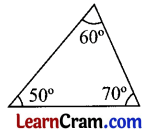Solution:
Acute angled triangle

(b)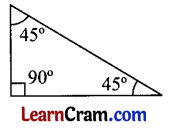Solution:
Right triangle

(c)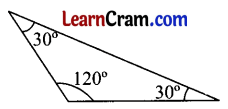Solution:
Obtuse triangle

(d)Solution:
Acute angled triangleQuestion 5.
Find which of the following can be measured by three angles of a triangle.
(a) 45°, 90°, 45°
Solution:
45° + 90° + 45° = 180°
Yes, it can be a measure of the angle ∆.

(b) 65°, 30°, 10°
Solution:
65° + 30° + 10° = 105°
No, it cannot be measured by angle ∆.

(c) 60°, 40°, 80°
Solution:
60° + 40° + 80° = 180°
Yes, it can be a measure of the angle ∆.

(d) 75°, 35°, 45°
Solution:
75° + 35° + 45° = 155°
No, it cannot be measured by angle ∆.

Question 6.
Classify the following triangle as equilateral, acute, scalene, and isosceles triangle.
(a)Solution:
Equilateral triangle

(b)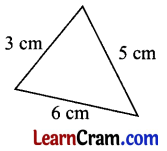Solution:
Scalene triangle

(c)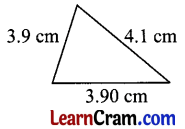Solution:
Isosceles triangle

(d)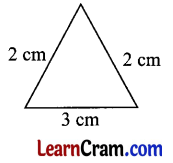Solution:
Isosceles triangle

(e)Solution:
Scalene triangleQuestion 7.
Find whether it is possible to draw a triangle with a line segment of length
(a) 12 cm, 7 cm, 8 cm
Solution:
12 cm, 7 cm, 8 cm
Sum of 2 sides = 12 cm + 7 cm = 19 cm
Third side = 8 cm
So 19 > 8 possible
Sum of 2 sides = 7 cm + 8 cm = 15 cm
Third side = 12 cm
15 cm > 12 cm possible
or
Sum of 2 side = 12 cm + 8 cm = 20 cm
Third side = 7 cm
20 cm > 7 cm possible
So triangle is possible.

(b) 2 cm, 3 cm, 9 cm
Solution:
2 cm, 3 cm, 9 cm
Sum of two side = 2 cm + 3 cm = 5 cm
Third side = 9 cm
5 cm < 9 cm So, the triangle is not possible.

(c) 20 cm, 17 cm, 18 cm
Solution:
20 cm, 17 cm, 18 cm
Sum of 2 sides = 20 cm + 17 cm = 37 cm
Third side = 18 cm
So 37 > 18 possible
Sum of 2 sides = 17 cm + 18 cm = 35 cm
Third side = 20 cm
35 cm > 20 cm possible
or
Sum of 2 side = 20 cm + 18 cm = 38 cm
Third side = 17 cm
38 cm > 17 cm possible
So triangle is possible.

(d) 2.3 cm, 3.2 cm, 6.6 cm
Solution:
2.3 cm, 3.2 cm, 6.6 cm
Sum of two side = 2.3 cm + 3.2 cm = 5.5 cm
Third side = 6.6 cm
5.5 cm < 6.6 cm
So, the triangle is not possible.

Question 8.
Identify the scalene, isosceles, and equilateral triangles.
(a)Solution:
Equilateral triangle.

(b)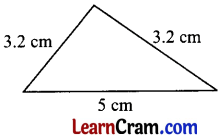Solution:
Isosceles triangle

(c)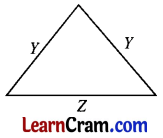Solution:
Isosceles triangle

(d)Solution:
Scalene triangleQuestion 9.
Find the third angle of this triangle. What type of triangle is this?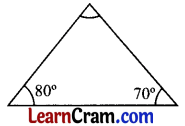Solution:
Let the third angle be x°
80° + 70° + x° = 180° (sum of angles of Δ is 180°)
⇒ 150° + x° = 180°
⇒ x = 70°
∴ 80°, 70°, 70°
The triangle is an acutely angled triangle.

Question 10.
If the measure of one angle of a right triangle is 60°. Find the third angle.
Solution:
As it is a right-angled triangle one of the angles is 90°.
Other angle = 60°
Let the third angle be x°
x° + 90° + 60° = 180°
⇒ x° + 150° = 180°
⇒ x° = 180° – 150°
⇒ x° = 30°
So, the other two angles are 90° and 30°.

Question 11.
The sum of two angles of a triangle is 100°. Find the third angle.
Solution:
Sum of two angles = 100°
Let the third angle = x°
x° + sum of two angles = 180° (sum of 3 angles of triangle is 180°)
⇒ x + 100° = 180°
⇒ x° = 180° – 100°
⇒ x° = 80°
The third angle is 80°.Question 12.
Identify the acute, obtuse, and right-angled triangle.
(a)Solution:
Acute angled triangle

(b)Solution:
Obtuse angled triangle

(c)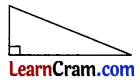Solution:
Right-angled triangle